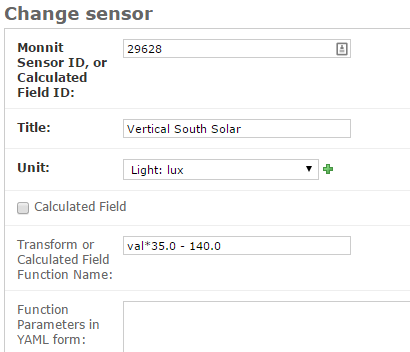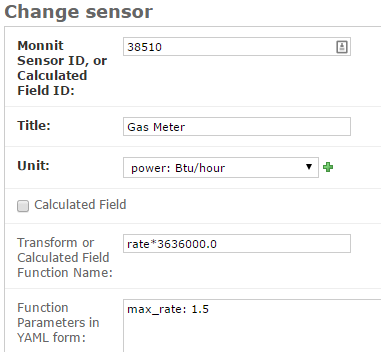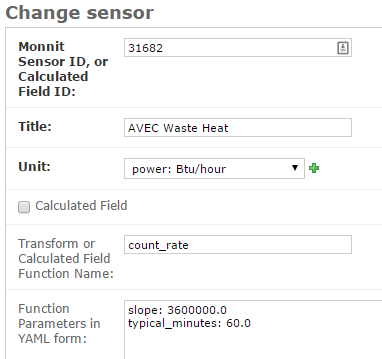# Transform Expressions¶

Transform Expressions are used for a number of purposes, including:

• Sensor values may arrive expressed in units that are not desirable. A Transform Expression can be used to convert the units to the type desired for display.
• Sensor values may have known errors that can be corrected with a Transform Expression before storage into the system.
• Pulse counts generally should be converted into a rate value (units/second or units/hour), as counts do not always arrive at equal intervals due to wireless transmission issues and due to users changing the time interval that a sensor reports. Special Transform Expressions can be used to convert the pulse counts into a rate value.

## Simple Transform Expressions¶

Transform Expressions are entered on the Sensor editing screen. The screenshot below shows a Transform Expression to adjust the reading from a Light Sensor, correcting for light reducing paint that covers the sensor.In the `Transform or Calculated Field Function Name` box you can see the transform expression `val*35.0 - 140.0`. The variable `val` is always available in a Transform Expression and it contains the raw sensor value that was posted. This transform multiplies that raw value by 35.0 and then subtracts 140.0. When creating these expressions, you have all the built-in functions (e.g. `abs()` for absolute value, ) from the Python programming language plus the functions available in the Python math module (e.g. `sqrt()`, `sin()`, `log10()`). So, an expression such as `sqrt(val)/(val - 8.6)` is a valid transform expression.

In the above screenshot, note that the `Calculated Field` box is not checked. This is not a new calculated field, created from other sensor values or the gathered from the Internet. Instead, this is just a conversion of an incoming sensor value.

## Pulse Counter Transforms¶

Electric meters, gas meters, water meters, and fuel meters often produce pulses as a means of electronically communicating flow through the meter. Each pulse represents a fixed amount of energy or volume of material flowing through the meter. The pulse output of the meter can be connected to a pulse counter that reports total pulse counts to the BMON system. It is generally desirable to convert these pulse count totals into a rate of flow of energy, fluid, or gas. By converting to a rate (units/second), variations in the length of the interval measured by the pulse counter are factored out of the measured value.

Special pulse counter Transform features were developed to address this situation. The recommended setting for a pulse counter is to have it continually accumulate pulse counts, only resetting to zero when a maximum rollover pulse count is reached. If your pulse counter is set up in this way, below is an example of how you can transform the total pulse count values into a usable rate value. In this example, a natural gas meter is being read, and a pulse occurs for each CCF of gas passing through the meter. Our objective is to convert the pulse count readings into a gas flow measured in Btu/hour.The BMON Transform feature has a special variable named `rate`. If a Transform expression uses this variable, BMON knows that a pulse count is being measured, and BMON automatically calculates the pulse rate per second indicated by the last pulse count reading received relative to the prior pulse count reading. This pulse rate is stored in the variable `rate`. As an example, assume that the last pulse count received was a total count of 10,435. The prior pulse count reading from the sensor was 9,623 and it was received 605 seconds prior to the current reading. The pulse rate that occurred between the two readings was `(10435 - 9623) / 605 = 1.342 pulses/second`. This value of 1.342 is automatically stored in the `rate` variable.

So continuing with the example, we know that BMON has already calculated pulse rate per second for the gas meter. We also know that one CCF of gas contains 1,010 Btus. To convert the pulse rate into Btus/hour we would do the following:

```rate (pulses/sec)  *  1,010 Btus/pulse  *  3,600 sec/hour

which is rate * 3636000
```

`rate * 3636000` is the expression shown in the `Transform or Calculated Field Function Name` box.

There are couple other optional but often important parameters that can be entered in the `Function Parameters in YAML form` box:

`max_rate` (default value = 5.0, expressed as pulses/second): Pulse count reporting errors (one common issue described below) can sometimes lead to an erroneous high pulse rate calculation. BMON will not save any sensor values if the pulse rate is above this `max_rate` value. For the example above, a maximum pulse rate 1.5 pulses per second is set. This corresponds to a 5,454,000 Btu/hour rate. Reported sensor values will be rejected if they exceed this rate. Note that there is a default `max_rate` value of 5.0 pulses/second if you do not provide a value in the `Function Parameters` box.

`rollover` (default value = 65536, largest 16 bit value): Pulse counters usually have a maximum pulse count that they record before rolling over to zero. BMON will account for this rollover when calculating the pulse rate. Year 2014 and prior Monnit wireless pulse counters roll over at a count of 65,536, the default value for this parameter. Newer Monnit pulse counters are 32 bit and roll over at 4,294,967,296. If you expect your pulse counter to eventually roll over, you need to ensure that BMON is using the correct `rollover` value.

`ignore_zero` (default value = `True`, the other valid value is `False`): Monnit Wireless pulse counters occasionally reset to a pulse count of zero if there are transmission problems or an accidental reset of the sensor. So, the pulse count value of zero is usually an erroneous value. By leaving this `ignore_zero` parameter at its default value of `True`, these zero pulse count readings will be ignored and no value will be stored until the next valid reading.

`ignore_negative` (default value = `True`, the other valid value is `False`): If set to `True` a backward movement of the counter is ignored (it could be due to a reset). If this parameter is `False`, a backward movement of the counter is reported as a negative rate of change.

`min_interval` (default value = 60, measured in seconds): Monnit wireless pulse counters sometimes send the same pulse count value twice but with slight separation in time, resulting in a zero calculated pulse rate. To filter out these erroneous readings you can set a `min_interval` between valid readings, measured in seconds. If two successive readings are separated by less than this number of seconds, the second reading will be rejected. The default value is 60 seconds.

Also note that all readings that use this pulse count transform are time-stamped at the midpoint between the current reading time and the previous reading time, since the rate reported is derived from the interval spanning those two time points.

If your pulse counter is setup to reset to zero after it reports its pulse count (Monnit wireless pulse counters 2013 and earlier), a different Transform function must be used to convert the counter values into rates. This is not the preferred method for setting up a pulse counter but may be needed for those counters that do not have a count accumulation mode, as described in the prior section.

The screenshot below is an example of configuring a counter that resets to zero after each sensor report.The text `count_rate` must be entered into the `Transform or Calculated Field Function Name` box. Then, additional parameters are entered into the `Function Parameters in YAML form` box. Each parameter has a default value, so they are not required to be entered, but you will likely need to override some of the default values.

`slope` (default value = 1.0) `offset` (default value = 0.0): BMON will automatically convert the incoming pulse count into a rate of pulses per second. The `slope` and `offset` parameters are used to convert this pulse rate into the desired engineering units, such as Btu/hour or kW. The final value stored in the sensor database is: `(pulse rate per second) * slope + offset`

`typical_minutes` (default value = 30.0, measured in minutes): Sensor transmissions are sometimes missed or occur multiple times due to poor signal strength. The BMON attempts to correct some of these problems but needs to know what the typical spacing is between sensor transmission. Enter that value expressed in minutes for this parameter.

`no_zero_after_link` (default value = `True`, other possible value is `False`): If a Monnit wireless sensor is having difficulty communicating with its gateway, it will sleep for two hours and then try to reestablish contact. When it does this, it will send an initial count of zero. By setting `no_zero_after_link` to `True` (the default value), these zero readings will not be stored in the sensor database.